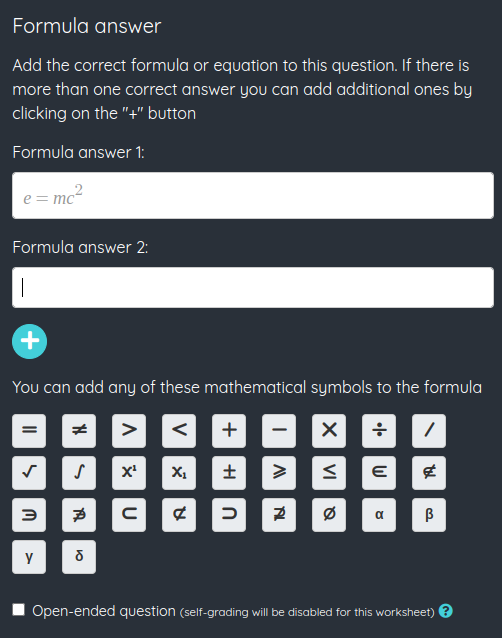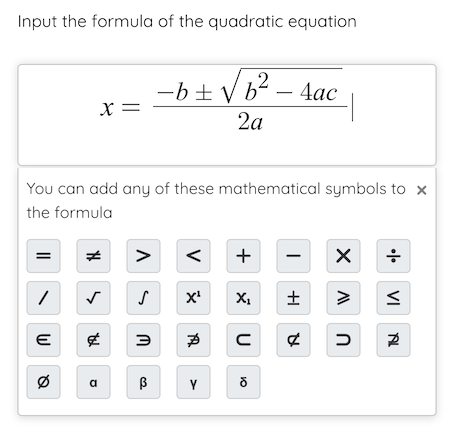The Formula answer fields must be filled in by the student, as the Written answer fields, but in this case they can input a formula or an equation. To make this easier, a panel will appear besides the field with a series of mathematical symbols to  input them.

We have two possibilities when adding this type of field: closed questions and open questions. The closed questions will have one or more correct answers (in case a formula or equation can be written in different ways) that we have to input when creating the field, and can be autocorrected. The open questions do not have a specified correct answer, the teacher will have to correct the question once the student finishes the worksheet.Once a Formula answer field has been added, its options screen will appear. We will have to input the correct answer or answers, and all of them would be counted as valid when autocorrecting the worksheet.

To add more options to the correct answer we must click the + button. We can also add new options with the keyboard, by pressing Ctrl+Intro (or ⌘+Intro in Mac). The editor will display the field filled in with the first of the correct answers.

If we mark the open-ended check this will be an open-ended question and we won't be able to add any answers, also the worksheet won't be self-graded and the question will have to be corrected manually.« Previous
Next »